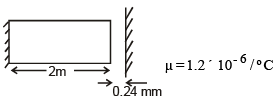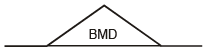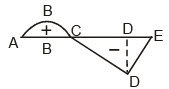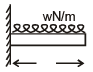# Test: Strength of Materials - 2

## 25 Questions MCQ Test Mechanical Engineering SSC JE (Technical) | Test: Strength of Materials - 2

Description
Attempt Test: Strength of Materials - 2 | 25 questions in 50 minutes | Mock test for Mechanical Engineering preparation | Free important questions MCQ to study Mechanical Engineering SSC JE (Technical) for Mechanical Engineering Exam | Download free PDF with solutions
QUESTION: 1

Solution:
QUESTION: 2

Solution:
QUESTION: 3

### The maximum deflection of a simply supported beam due to its self weight (W) is.

Solution:
QUESTION: 4

It flexural strength decreases the maximum slopeof any beam

Solution:
QUESTION: 5

If temperature of a freely hanging bar is increasedit will develope : -

Solution:
QUESTION: 6

Find the increase in temperature (DT) so that the gap gets filledSolution:
QUESTION: 7

The ratio of hoop stress for a thin cylinder andthin spherical pressure vessel is

Solution:
QUESTION: 8

A spherical pressure vessel is to be designed tohold 40 MPa pressure and Diameter should be200 mm. If max stress can be 200 MPa find thethickness required.

Solution:
QUESTION: 9

Shown below is a B.M.D.The SFD will be

Solution:
QUESTION: 10

Shown is a B.M.DThe shear force is zero at

Solution:
QUESTION: 11

Shown is a B.M.DIn the above diagram point of contraflexure is

Solution:
QUESTION: 12

In the above shown BMD, the beam is mostprobably

Solution:
QUESTION: 13

Strain Energy for the shown beam isSolution:
QUESTION: 14

The B.M.D. for a cantilever beam due to its selfweight is

Solution:
QUESTION: 15

For a structural member the principle stressesare equal but opposite in nature . The center ofmohr's circle will

Solution:
QUESTION: 16

The maximum shear stress in a mohr's circle isequal to

Solution:
QUESTION: 17

A spring of length 'L' is cut into 5 equal parts if initial spring stiffness was R the final stiffness after
parallel combination of all 5 parts will be

Solution:
QUESTION: 18

It E is the young's Modulus, the minimum value of bulk modulus is

Solution:
QUESTION: 19

It the dimentions of a prismatic bar hanging fromroof are doubled, the elongation due to its selfweight will become

Solution:
QUESTION: 20

A long shaft of diameter d is subjected to twisting moment of T. The maximum normal stress acting
at the cross- section is equal to :-

Solution:
QUESTION: 21

For shafts in parallel which of the following willbe same for all shafts.

Solution:
QUESTION: 22

How many minimum values among E, G, K, Mwould you require for a isotropic andhomogenous material to determine all state ofstress.

Solution:
QUESTION: 23

The maximum shear stress in a shaft of Diameter100 mm is 100MPa. The shear stress at adistance of 30 mm from center is .

Solution:
QUESTION: 24

The depth of a beam of uniform strength vary : -

Solution:
QUESTION: 25

Which of the following is must for a strut.

Solution:Use Code STAYHOME200 and get INR 200 additional OFF Use Coupon Code